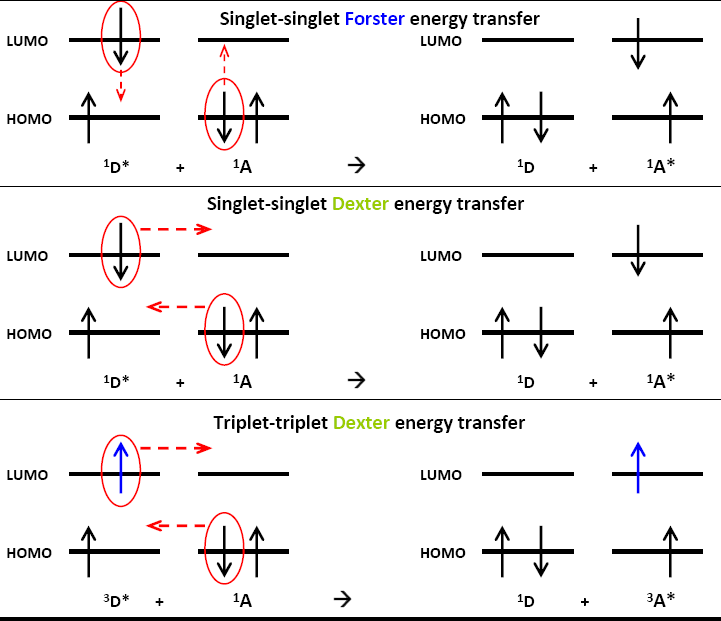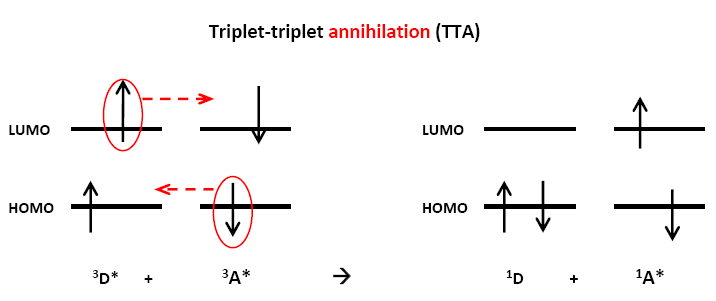# Dexter Energy Transfer

$$\newcommand{\vecs}{\overset { \rightharpoonup} {\mathbf{#1}} }$$ $$\newcommand{\vecd}{\overset{-\!-\!\rightharpoonup}{\vphantom{a}\smash {#1}}}$$$$\newcommand{\id}{\mathrm{id}}$$ $$\newcommand{\Span}{\mathrm{span}}$$ $$\newcommand{\kernel}{\mathrm{null}\,}$$ $$\newcommand{\range}{\mathrm{range}\,}$$ $$\newcommand{\RealPart}{\mathrm{Re}}$$ $$\newcommand{\ImaginaryPart}{\mathrm{Im}}$$ $$\newcommand{\Argument}{\mathrm{Arg}}$$ $$\newcommand{\norm}{\| #1 \|}$$ $$\newcommand{\inner}{\langle #1, #2 \rangle}$$ $$\newcommand{\Span}{\mathrm{span}}$$ $$\newcommand{\id}{\mathrm{id}}$$ $$\newcommand{\Span}{\mathrm{span}}$$ $$\newcommand{\kernel}{\mathrm{null}\,}$$ $$\newcommand{\range}{\mathrm{range}\,}$$ $$\newcommand{\RealPart}{\mathrm{Re}}$$ $$\newcommand{\ImaginaryPart}{\mathrm{Im}}$$ $$\newcommand{\Argument}{\mathrm{Arg}}$$ $$\newcommand{\norm}{\| #1 \|}$$ $$\newcommand{\inner}{\langle #1, #2 \rangle}$$ $$\newcommand{\Span}{\mathrm{span}}$$$$\newcommand{\AA}{\unicode[.8,0]{x212B}}$$

Dexter energy transfer is sometimes called short-range, collisional or exchange energy transfer which is a non-radiative process with electron exchange. Dexter Energy transfer although similar to Förster energy transfer, differs greatly in length scale and underlying mechanism.

## Introduction

The energy transfer could take place via the interaction between an excited chemical group, say D*, and a ground-state chemical group, say A, without emitting a photon when transferring energy. Förster provided a model saying that the energy released from an excited donor could simultaneously excite the ground-state acceptor based on the Coulombic interaction between these two chemical groups. Independently, David L. Dexter provided another mechanism that an excited donor group and an acceptor group might indeed exchange electrons to accomplish the non-radiative process. The Dexter exchange energy transfer is generally associated with quenching. The term “quenching” means any physical process or molecular state that decreases the molecular fluorescence. Previously, the Dexter energy transfer was treated a fundamental phenomena in photochemistry field. Recently, it is greatly applied to novel emitting materials, such as white organic light-emitting diodes and energy up-conversion systems. (Blue light emission and white light emission.

## The big picture of Dexter Energy Transfer

Dexter energy transfer is a process that two molecules (intermolecular) or two parts of a molecule (intramolecular) bilaterally exchange their electrons. Unlike the sixth-power dependence of Förster energy transfer, the reaction rate constant of Dexter energy transfer exponentially decays as the distance between these two parties increases. On account of the exponential relationship to the distance, the exchange mechanism typically occurs within 10 Angstroms. Hence, the exchange mechanism is also called the short-range energy transfer.Figure $$\PageIndex{1}$$: Schematic diagram for Förster and Dexter energy transfer.

The exchange mechanism is based on the Wigner spin conservation rule; thus, the spin-allow process could be:

Singlet-singlet energy transfer: $\ce{^{1}D^{*} + ^1A -> ^{1}D + ^{1}A^{*}}$

It could be understood by: a singlet group produces another singlet group.

Triplet-triplet energy transfer: $\ce{^{3}D^{*} + ^{1}A → ^{1}D + ^{3}A^{*}}$

It could be understood by: a triplet group produces another triplet group.

The singlet-singlet energy transfer can happen when undergoing the Coulombic interaction. However, the Coulombic interaction will not involve the triplet-triplet energy transfer because that violates the Wigner spin conservation law.

## Important factors about Dexter Energy transfer

### Wavefunction Overlap

As the figure 1 implies, the Dexter energy transfer is a process that the donor and the acceptor exchange their electron. In other words, the exchanged electrons should occupy the orbital of the other party. Hence, besides the overlap of emission spectra of $$D$$ and absorption spectra of $$A$$, the exchange energy transfer needs the overlap of wavefunctions. In the popular words, it needs the overlap of the electron cloud. The overlap of wavefunctions also implies that the excited donor and ground-state acceptor should be close enough so the exchange could happen. If $$D$$ and $$A$$ are different molecules, collisions can greatly make them bump into each other. The short distance that makes energy transfer happen is almost comparable to the collisional diameter. This is the reason why the exchange energy transfer is always referred to the term “collision.”

### Rate constant

The rate constant of exchange energy transfer is given by

$k_{dexter}= KJ \; \text{exp} \left (\dfrac {-2R_{DA}}{L} \right )$

while the rate constant of exchange energy transfer is given by

$k_{dexter} \propto \dfrac {D_{D}^{2} D_{A}^{2}}{R_{DA}^{6}}$

$$J$$ is the normalized spectral overlap integral. The term “normalized” here means making the absorption spectra and the emission spectra on the same scale and have the same highest level (For real examples, please refer to this paper) $$K$$ is an experimental factor, $$R_{DA}$$ is the distance between $$D$$ and $$A$$, and $$L$$ is the sum of van der Waals radius; $$D_D$$ is the transition dipole of donor, $$D_A$$ is the transition dipole of acceptor. Comparing rate constants of different energy transfer models, we can find that the rate constant of exchange energy transfer decays steeply because of its intrinsic exponential relationship, which is the reason that the exchange energy transfer is also called the short-range energy transfer and the Förster mechanism is called the long-range energy transfer.

### Preparing the excited triplet state of interest

According to the Figure $$\PageIndex{1}$$, by exchanging electrons, a triplet excited donor could also excite the acceptor to its triplet state. This process is called “sensitization” process; hence, the triplet excited-state donor is the sensitizer. Generally speaking, the time scale for fluorescing a photon is about several nanosecond. The intersystem crossing happens in this time scale, too. However, roughly speaking, fluorescence generally happens faster that the intersystem crossing, which means an electron would fluoresce before it proceeds to occupy a triplet state through thermal relaxation.

### A special case: triplet-triplet annihilationFigure $$\PageIndex{2}$$: Schematic diagram for triplet-triplet annihilation.

The triplet-triplet annihilation (TTA) is an important case of exchange energy transfer. Two triplet chemical groups, D* and A*, react to produce two singlet states. Generally, the energy gap between S0 and T1 is bigger than the energy gap between T1 and S1. Hence, if two triplet excited-state molecules encounter, the process might have enough energy to excite one of them to the higher singlet states, which can make the fluorescence happen in this system. After the annihilation process, the energy level that the electron occupies will be twice of the lowest triplet energy gap.Figure $$\PageIndex{3}$$: Schematic diagram for triplet-triplet annihilation.

## Conclusion

The Dexter energy transfer is a fundamental phenomenon in photochemistry. The difference between Förster and Dexter mechanism include:

1. Dexter mechanism involve the overlap of wavefunctions so that electrons can occupy the other’s molecular orbitals.
2. The reaction rate constant of Dexter energy transfer sharply decreases while the distance between $$D$$ and $$A$$ increase and the distance is generally smaller than 10 angstroms.
3. The Dexter mechanism can be applied to produce the triplet state of some molecules of interest.
4. The special case of exchange-triplet-triplet annihilation-can “push” the electron to upper singlet states by exchanging the electrons of two triplet molecules.

## ProblemsThe figure above is the energy levels that stands for an upconversion fluorescence system. Surprisingly, the Dexter energy transfer and the triplet-triplet annihilation mechanisms provide a fantastic way to produce high-energy light simply by using medium-energy light. If choosing the donor and the acceptor properly, one can produce three kinds of wavelengths of fluorescence, say red, green, and blue. After mixing these light, this system might produce white light if the ingredient light is well mixed. Today, one can even simply predict th photophysical properties by using commercial quantum chemistry software, before starting to do the experiment. Below are some questions behind this figure.

## Problems

(These question are adapted from Chemical Communication, 2009, 27, 4064 and Physical Review B, 2008, 78, 195112)

Question 1: What does the worm-like arrow "A" stand for?

Question 2: What does the worm-like arrow "B" stand for?

Question 3: What does the process "C" stand for?

Question 4: What does the process "D" stand for?

Question 5: The original compound absorbs the light with frequency v1 and ultimately emits the light

with frequency v2. Which frequency is bigger? So this research group used low-energy

light to produce high-energy light or use inverse idea? Does it make sense?

Answer 1: Internal conversion or vibrational relaxation.

Answer 3: Dexter exchange energy transfer.